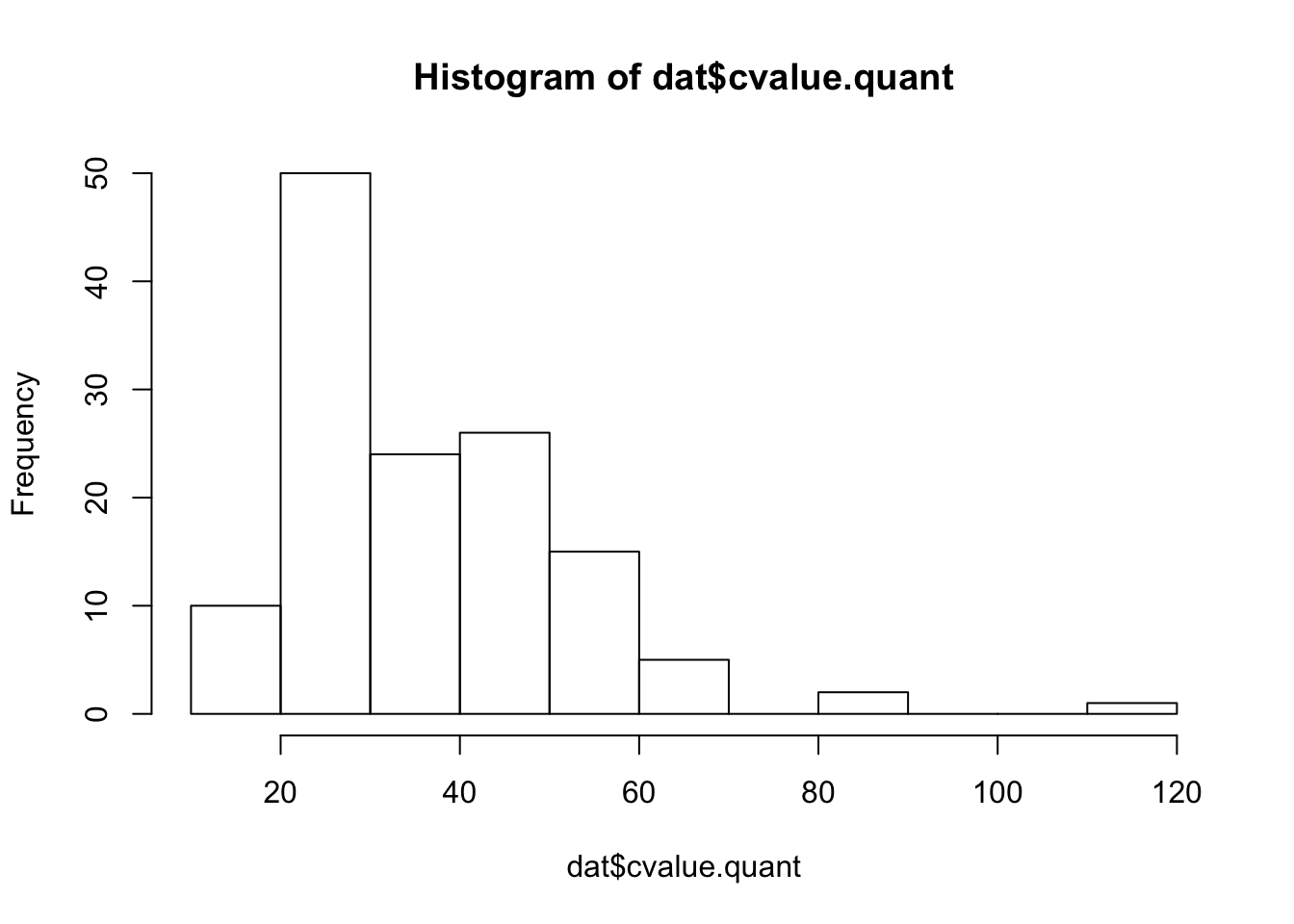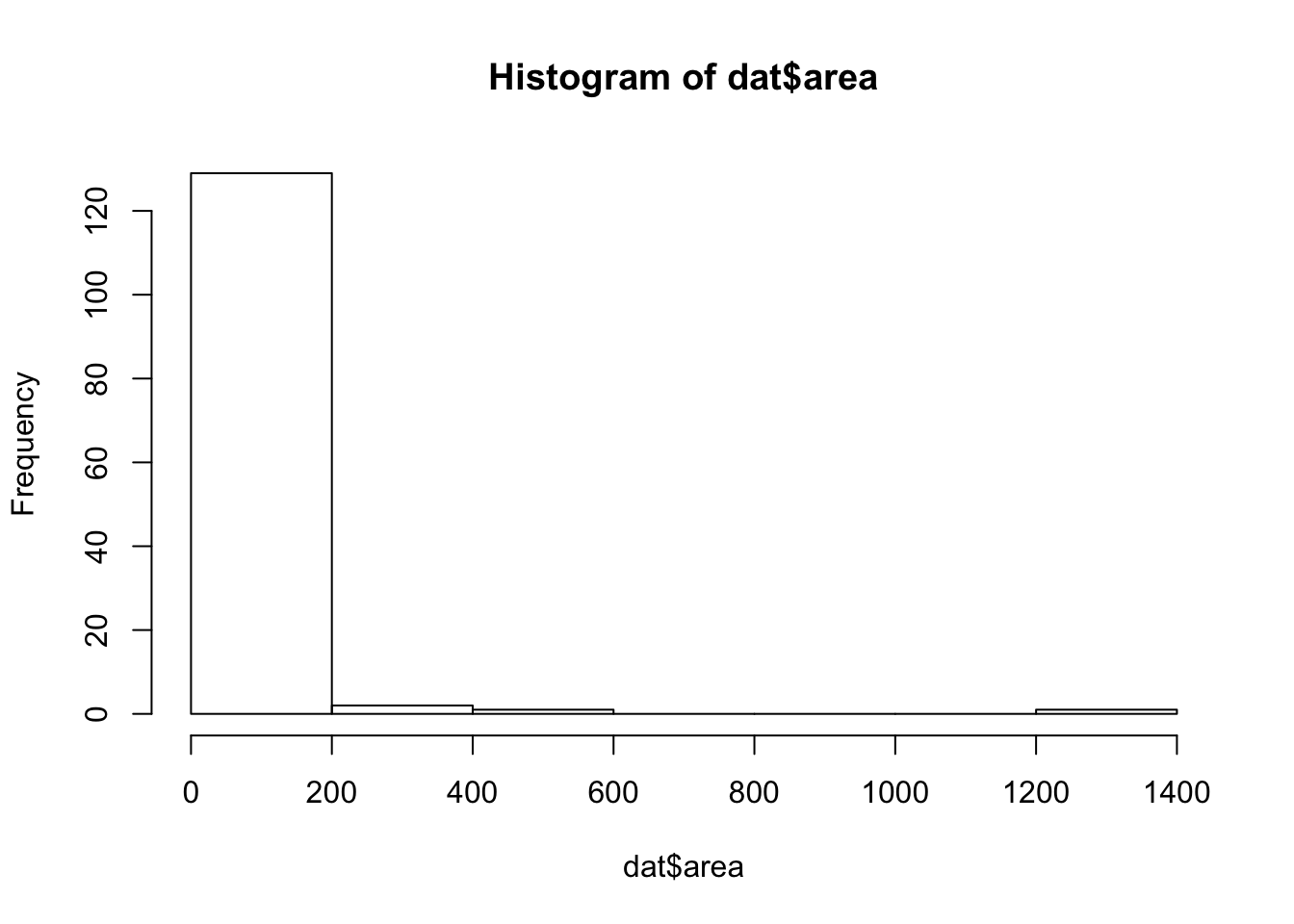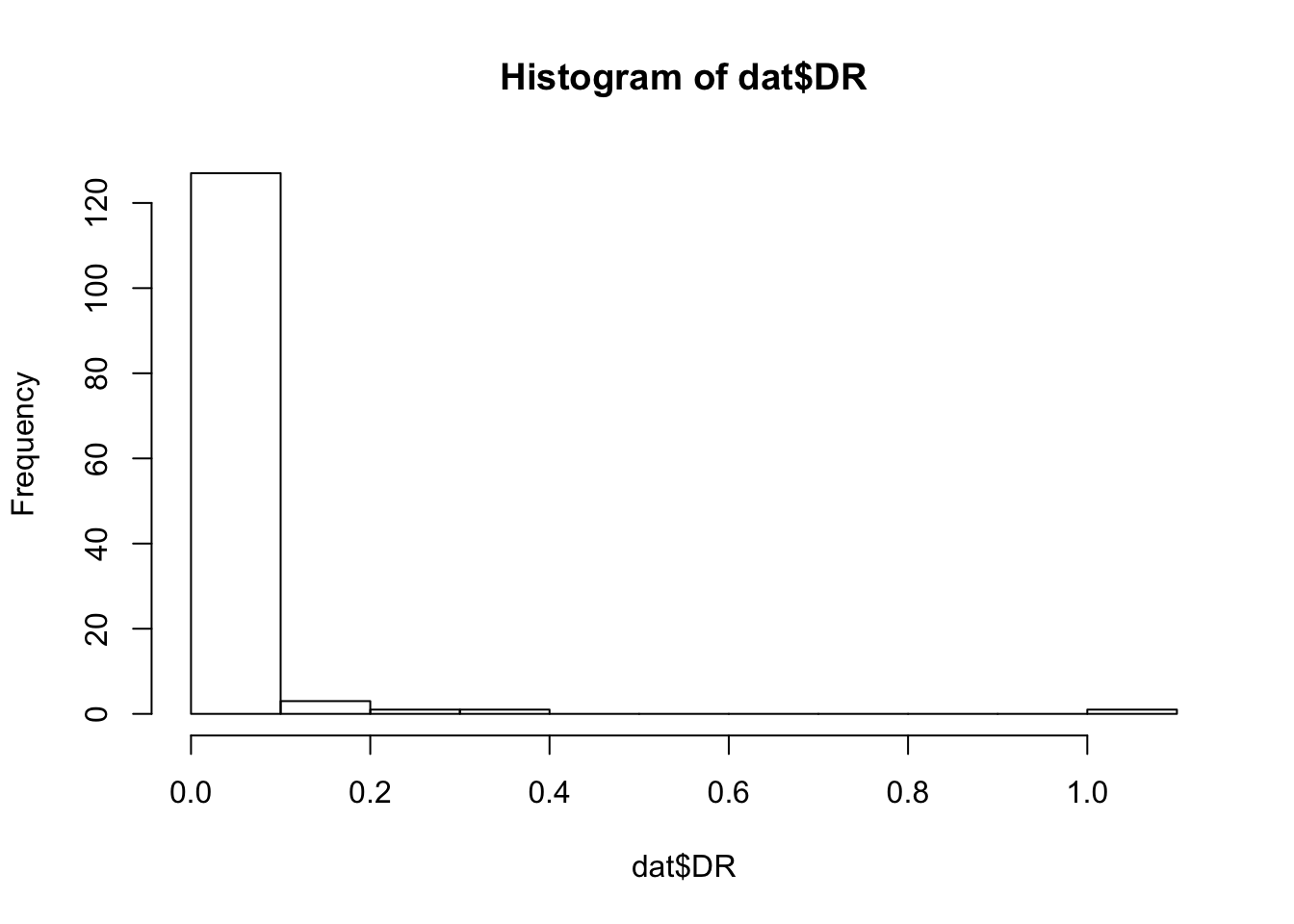#### These relationships are illustrated below.#### From this, we can come up with a series of simple linear models, excluding any interaction terms.

##### Load the Data
``````setwd('~/Dropbox/Phylo_Comp_Meth/')
tree <- read.nexus('caud.ultra.nexus')
dat <- read.csv('Caudata_PhyloComp_Data.csv')
rownames(dat) <- dat\$Species
dat <- dat[,c(1,8,10,13,14,15,16)]
dat <- na.omit(dat)

# Because these are raw data, we need to do a few things first. This includes converting latitude to absolute value (we are interested in the effects of distance from the equator), and normalizing non-normal data.
# Convert latitude to absolute latitude.
dat\$lat <- abs(dat\$lat)

# Lets look at the normality of our data.
hist(dat\$cvalue.quant)````````hist(dat\$area)````hist(dat\$DR)````hist(dat\$Abs.Mol.Rate)``